# How to Generate Random Numbers in Excel (Without Duplicates)

Randomness is often just as necessary as order and symmetry, whether you are dealing with life or an Excel worksheet. Sometimes, you need it to ensure every value has an equal opportunity, like in a lottery.

The concept of ‘chance’ also plays an important role in simulating complex systems. It helps you test data. We often use random numbers to generate sample datasets for our tutorials too!

The good news is, Excel contains some very useful functions to help generate random numbers. The bad news is that these functions don’t always promise a unique set of random numbers.

If you look up ‘How to Generate Random Numbers without Duplicates in Excel’, you’ll probably find at least 10 different ways to do it.

Most of them work quite well too. However, we’ve noticed most tutorials leave you with a really long formula and hardly any explanation on how it works.

In this tutorial, we will show you 2 different ways in which you can generate unique random numbers in Excel using formulas.

## Random Number Generating Functions in Excel

There are mainly 2 random number generating functions available in Excel. Let us see them one by one:

### RANDBETWEEN Formula

The RANDBETWEEN function is a great function that helps you generate random whole numbers between an upper and lower limit.

The syntax for this function is:

`=RANDBETWEEN([lower_limit],[upper_limit])`

In the above formula, you can specify the lower and the upper limit. For example, if the limits are 10 and 1000, this formula will generate random numbers between these two specified limits.

This method most likely produces unique results if you have a larger range and need to generate a small number of random numbers.

For example, if you need to generate 10 numbers in the range of 10 to 1000. However, this is not the most reliable method for getting unique random numbers.

### RAND Formula

The RAND function returns a decimal random number between 0 and 1. It takes no arguments, so its syntax is just

`=RAND()`

The great thing about this function is that there is a good probability that the numbers generated by it will not repeat, at least for 100,000 times.

The main differences between RAND() and RANDBETWEEN() are :

• RANDBETWEEN returns a whole number while RAND returns a decimal number
• RANDBETWEEN needs two parameters that specify the range in which the random numbers will be generated. RAND does not take any parameters. It can generate any number between 0 and 1.
• RANDBETWEEN is less likely than RAND to generate unique random numbers.

Both RANDBETWEEN and RAND recalculate values every time the worksheet gets updated.

That means you get a new random number every time you change any cell value in the worksheet or press the F9 key.

Both these numbers fail to provide truly random numbers if used on their own, but combining them with other functions, gives a high probability of getting random numbers without repetition. Let us see how.

## Using RAND and RANK to get Random Numbers in Excel Without Duplicates

You can combine the RAND and RANK functions to get a set of random numbers without duplicates.

The RANK function returns the rank of a number within a set of numbers.

Its syntax is as follows:

`=RANK(number, array,[order])`

The first parameter is the number or reference that you want to rank.

The second parameter is the set of numbers or range of cells that you will rank from.

The third parameter is optional. It specifies whether you want to rank in ascending or descending order. If it’s 0, the numbers are ranked in descending order. If otherwise the numbers are ranked in ascending order. By default, the numbers are ranked in descending order.

Let’s say you want 10 random numbers from A2 to A11. Here’s how you can combine the two functions:

1. In the first cell (A2), type: =RAND(). This will generate a random number between 0 and 1.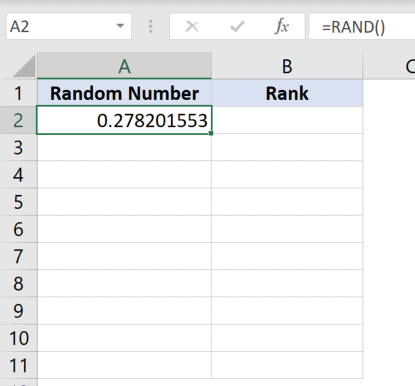2. Pull down the fill handle (located at the bottom right corner of the cell) to copy the formula to as many cells as you need. So if you want 10 random numbers, copy it down to cell A11.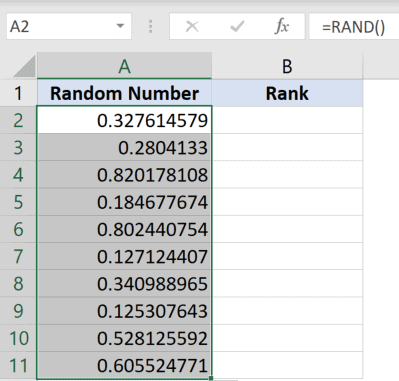3. In the adjacent column (B), use the RANK formula as follows: =RANK(A2, \$A\$2:\$A\$11). The second parameter is a range of absolute cell locations. These make sure the cell references do not change when the formula is copied to other cells.
4. Press the Return Key.
5. Drag down the fill handle for the cell (B2) or just double click it.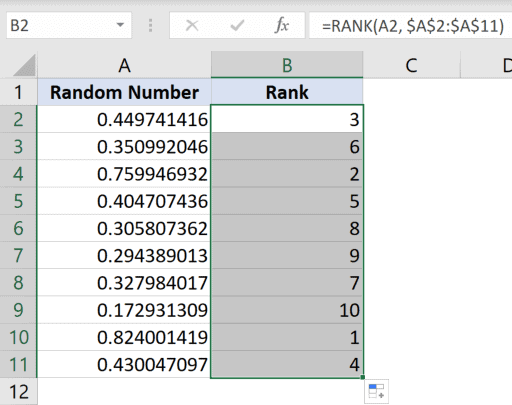6. Now, these numbers are volatile at the moment, since the RAND() function always recalculates every time there’s a change in the worksheet. To avoid that, copy the cells (B2:B11) and paste them in the same place as value.
7. Delete the first column (A).

This should give you a list of 10 random numbers, and that too without any repetition.

All we did here is get a random set of decimal numbers between 0 and 1 and then rank these numbers from 1 to 10 to get unique random integers.

Thus, we got a nice random set of numbers between 1 and 10 in column B (before deleting Column A).

This method should give you completely random and unique numbers with 99% assurance. But there might be slight discrepancies if you have to generate a much larger set of numbers.

For this, you can use the Remove Duplicates option to get rid of any duplicates.

Select the range of cells containing your random set of numbers.

From the Data tab, select Remove Duplicates from the Data Tools group.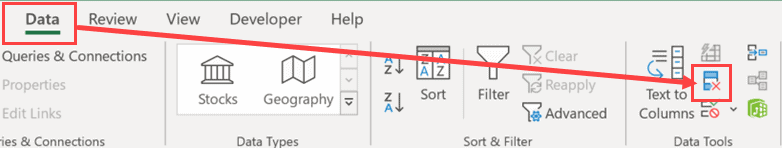## Using RAND, LARGE and MATCH to get Random Numbers in Excel Without Duplicates

A similar method is to use RAND in combination with LARGE and MATCH functions. The LARGE function returns the kth largest value in a list, for example, the fourth largest value or the fifth largest value, etc. The syntax for this function is

`=LARGE(array, k)`

Here, the first parameter is the set of numbers or range of cells in which you want to find the largest

The second parameter is an integer that specifies the position from the largest value, i.e. the kth position.

The MATCH function looks for an item in a range of cells and then returns the relative position of that item. The syntax for this function is:

`=MATCH(value, array, [match_type])`

Here, the first parameter is the value you are looking for.

The second parameter is the set from which you want to find the value’s position

The third parameter is optional. It is basically used to specify the type of matching that should be done (the largest value that is less than, the smallest value that is greater than or exact match).

Let’s say you want 10 random numbers from A2 to A10. Here’s how you can combine the three functions:

1. In the first cell (A2), type: =RAND(). This will generate a random number between 0 and 1.
2. Pull down the fill handle (located at the bottom right corner of the cell) to copy the formula to as many cells as you need. So if you want 10 random numbers, copy it down to cell A11.
3. In the adjacent column (B), use the LARGE formula as follows: =LARGE(\$A\$2:\$A\$11,ROW(A1)). The first parameter is a range of absolute cell locations. These make sure the cell references do not change when the formula is copied to other cells. Here, we are trying to find the 1st largest value from A2 to A11. ROW(A1) means the row number of A1, in other words, the number 1. This formula could also have been written as: =LARGE(\$A\$2:\$A\$11,1). But since we want the value to change when it is copied in each row, we used ROW(A1) instead.
4. Press the Return Key
5. Drag down the fill handle for the cell (B2) or just double click it.
6. In the next column (C), use the MATCH function as follows: =MATCH(B2,\$A\$2:\$A\$11,0). Here, we are trying to find the position of the value in B2 in the range A2 to A11. We want an exact match, so we specify the third parameter as 0.
7. Drag down the fill handle for the cell (C2) or just double click it.
8. These numbers are volatile at the moment since the RAND() function always recalculates every time there’s a change in the worksheet. To avoid that, copy the cells (C2:C11) and paste them in the same place as value.
9. Delete the first two columns (A and B).

This should give you a list of 10 random numbers without any repetition.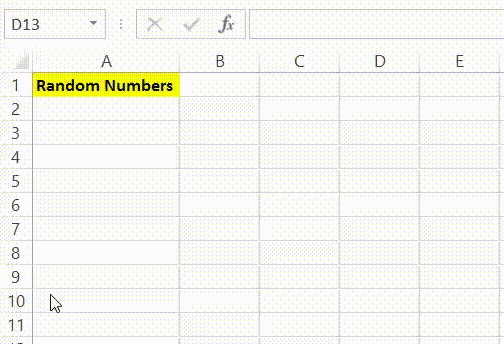In this method, we got a random set of decimal numbers between 0 and 1 in column A and then arranged them in descending order in column B.

Next, we found the position of each number from column B in the set of random numbers of column A. This gave us a random set of numbers between 1 and 10 in column C (before deleting Columns A and B).

All we did here was convert the random decimal numbers to their integer counterparts.

In this tutorial, we showed you two ways in which you can generate random numbers in Excel without repetition.

There are plenty of other ways to do this. We leave it up to you to decide which way you find the easiest and most effective.

Other Excel tutorials you may find useful: